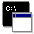## Firefly and PC GAMESS-related discussion club

Learn how to ask questions correctlyRe^9: Is it possible to get UV-VISIBLE AND RAMAN SPECTRUM USING FIREFLY???

Siddheshwar Chopra
sidhusai@gmail.com

Dear Thomas,
It was a detailed information provided from you. Thanks for that. I ran the RUNTYP=RAMAN calculation by adding the \$HESS from PUNCH file. In some earlier post I had read that \$HESS group is taken from IRCDATA file. I hope my procedure is correct (in Windows)?
Sir, I noticed that \$HESS group was missing from one of the PUNCH files, even though I ran the same code in all my samples. Why this happened?? A sample code is below:

\$SYSTEM MEMORY=28757184 AOINTS=DIST TIMLIM=5295600 KDIAG=0 FASTF=.T. \$END
\$CONTRL INTTYP=HONDO ICUT=10 ITOL=30 RUNTYP=OPTIMIZE SCFTYP=RHF \$END
\$CONTRL DFTTYP=B3LYP COORD=UNIQUE NZVAR=50 \$END
\$CONTRL MAXIT=10000 MOLPLT=.T. PLTORB=.T. \$END
\$CONTRL ICHARG=0 MULT=1 \$END
! \$CONTRL EXETYP=CHECK \$END
\$BASIS GBASIS=N31 NGAUSS=6 \$END
\$STATPT OPTTOL=10E-8 NSTEP=200 METHOD=GDIIS HSSEND=.TRUE. \$END
\$SMP HTTFIX=.F. \$END
\$P2P P2P=.T. MXBUF=2048 DLB=.T. \$END
\$SCF DIRSCF=.T. FDIFF= .T. DIIS=.T. \$END Ā
\$ZMAT DLC=.T. AUTO=.T. \$END
\$SCF NCONV=8 \$END
\$GUESS GUESS=HUCKEL KDIAG=0 \$END
\$DATA

Regards,

On Tue Jun 18 '13 9:26pm, Thomas wrote
--------------------------------------
>Dear Siddheshwar,

>The system you would like to perform the TDDFT calculation on should have been minimized.

>Oscillator strengths relate directly to absorbance. When you would record an experimental molar UV/vis spectrum (exc. coeff. in ĀL / mol cm vs frequency in Hz), integrate one of the absorption waves, and multiply this number by 1.44E-19, you would obtain the oscillator strength for that absorption. This can be found in many textbooks and even with a Google search.

>Linear response TDDFT only gives vertical excitation energies and their corresponding oscillator strengths. However, in an experimental spectrum each absorption has a certain broadness. LR-TDDFT unfortunately does not tell you how broad each absorption is. This is why the calculated spectrum is typically convoluted with Gaussian curves. How wide these curves should be is up to the user.
>
>
>Kind regards,
>Thom
>
>
>
>
>
>On Tue Jun 18 '13 8:34am, Siddheshwar Chopra wrote
>--------------------------------------------------
>>Dear Thomas,
>>Thanks for your reply. I have a doubt regarding the sample used for the calculation... How do I take the sample for UV-VISIBLE spectrum calculation?? I mean do I first GLOBALLY minimize it (by checking the Hessian)...and then run for RUNTYP=ENERGY under TDDFT??
>>I have downloaded the latest Gausssum version (2.2.6.1)..The plot is between, Oscillator strength (and Epsilon) Vs. Wavelength. How do I convert Oscillator strength to Absorbance??

>>Regards,

>>On Mon Jun 17 '13 12:59pm, Thomas wrote
>>---------------------------------------
>>>Dear Siddehshwar,

>>>I do not see any problems with your output file. Also, I am able to plot the UV/vis spectrum using GaussSum (version 2.2.6.1) without any problems, even when I set the range to 1 - 900 nm.

>>>There are a number of textbooks that provide an introduction into computational chemistry, and TDDFT is explained in most of them. To the best of my knowledge, oscillator strengths in Firefly are calculated using the formula on the page below:

>>>http://en.wikipedia.org/wiki/Oscillator_strengthhttp://en.wikipedia.org/wiki/Oscillator_strengthhttp://en.wikipedia.org/wiki/Oscillator_strengthhttp://en.wikipedia.org/wiki/Oscillator_strengthhttp://en.wikipedia.org/wiki/Oscillator_strengthhttp://en.wikipedia.org/wiki/Oscillator_strengthhttp://en.wikipedia.org/wiki/Oscillator_strengthhttp://en.wikipedia.org/wiki/Oscillator_strengthhttp://en.wikipedia.org/wiki/Oscillator_strengthhttp://en.wikipedia.org/wiki/Oscillator_strengthhttp://en.wikipedia.org/wiki/Oscillator_strengthhttp://en.wikipedia.org/wiki/Oscillator_strengthhttp://en.wikipedia.org/wiki/Oscillator_strengthhttp://en.wikipedia.org/wiki/Oscillator_strengthhttp://en.wikipedia.org/wiki/Oscillator_strengthhttp://en.wikipedia.org/wiki/Oscillator_strengthhttp://en.wikipedia.org/wiki/Oscillator_strengthhttp://en.wikipedia.org/wiki/Oscillator_strengthhttp://en.wikipedia.org/wiki/Oscillator_strengthhttp://en.wikipedia.org/wiki/Oscillator_strengthhttp://en.wikipedia.org/wiki/Oscillator_strengthhttp://en.wikipedia.org/wiki/Oscillator_strengthhttp://en.wikipedia.org/wiki/Oscillator_strengthhttp://en.wikipedia.org/wiki/Oscillator_strengthhttp://en.wikipedia.org/wiki/Oscillator_strengthhttp://en.wikipedia.org/wiki/Oscillator_strengthhttp://en.wikipedia.org/wiki/Oscillator_strengthhttp://en.wikipedia.org/wiki/Oscillator_strengthhttp://en.wikipedia.org/wiki/Oscillator_strengthhttp://en.wikipedia.org/wiki/Oscillator_strengthhttp://en.wikipedia.org/wiki/Oscillator_strengthhttp://en.wikipedia.org/wiki/Oscillator_strength
>>>
>>>
>>>Kind regards,
>>>Thom
>>>
>>>
>>>
>>>
>>>On Mon Jun 17 '13 9:05am, Siddheshwar Chopra wrote
>>>--------------------------------------------------
>>>>Dear Thomas,
>>>>Thank you for a prompt help. As per your guidance, I had run the calculation for the same sample. I just added this command "COORD=UNIQUE NZVAR=12". But I could not get the UV-VISIBLE spectrum in GaussSum at all. It says that no peaks were found in this range. I even changed the range from 1-900nm and so on. Please find attached the o/p file. A quick help would be appreciated. Also, I would be glad if you could throw light on HOW FIREFLY calculates the UV-Vis spectra?? I understand that for calculating IR spectra, HESSIAN is needed (force constant matrix); but how is the UV-VISIBLE spectra calculated? Do I need any hessian here? Any weblink would be appreciated too.

>>>>Regards
>>>>
>>>>
>>>>On Sat Jun 15 '13 12:51pm, Thomas wrote
>>>>---------------------------------------
>>>>>Dear Siddehshwar,

>>>>>Below is a very basic input example for a TDDFT calculation on butadiene. Note that it lacks a few groups and keywords that one would normally use in a multiprocessor environment, such as the \$P2P group. If you would like to run a CIS or TDHF calculation, you should change the value of CITYP to CIS or TDHF and remove the DFTTYP variable. Also, you should change the name of the \$TDDFT group to \$CIS or \$TDHF (keywords for these groups are the same).

>>>>>

```\$CONTRL CITYP=TDDFT DFTTYP=PBE0 RUNTYP=ENERGY \$END
\$SYSTEM TIMLIM=525600 MEMORY=10000000 \$END
\$BASIS GBASIS=N31 NGAUSS=6 NDFUNC=1 \$END
\$TDDFT NSTATE=4 ISTSYM=0 ISTATE=1 \$END
CNH 2
C 6.0 0.5262888328 0.5113710450 0.0000000000
C 6.0 1.8213687868 0.2431548398 0.0000000000
H 1.0 0.1953148875 1.5374180614 0.0000000000
H 1.0 2.5595257633 1.0243655101 0.0000000000
63
H 1.0 2.1906083178 -0.7681578804 0.0000000000
\$END```

>>>>>Right now, not all available XC functionals can be used for TDDFT calculations. The following functionals can be used only:

>>>>>

`SLATER VWN1RPA SVWN1RPA B1LYP B88 VWN5 BVWN1RPA BHHLYP XPW91 PW91LDA SVWN5 B1PW91 GILL96 LYP BVWN5 B3PW91 XPBE96 CPW91 SLYP B3LYP1 OPTX CPBE96 BLYP B3LYP5 GLYP O3LYP1 OLYP O3LYP5 XLYP X3LYP BPW91 PBE1PW91PW91 PBE0 PBEPW91 PBE96`

>>>>>Finally, I should mention that it is possible to request the Tamm-Dancoff approximation to TDDFT by specifying

>>>>>

`\$TDDFT TDA=.T. \$END`

>>>>>In the output, you can find excitation energies and oscillator strengths which can be used to plot the UV/vis spectrum. Useful programs for plotting the UV/vis spectrum are GaussSum (freeware) and ChemCraft.

>>>>>Hope this helps.
>>>>>
>>>>>
>>>>>Kind regards,
>>>>>ThomThis message contains the 14 kb attachment [ sample.dat ]Thu Jun 20 '13 9:49amThis message read 1181 times